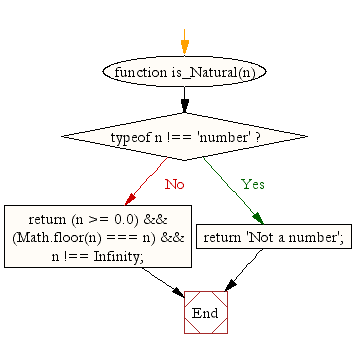# JavaScript: Find out if a number is a natural number or not

## JavaScript Math: Exercise-12 with Solution

Write a JavaScript function to find out if a number is a natural number or not.

Note:
Natural numbers are whole numbers from 1 upwards : 1, 2, 3, and so on ... or from 0 upwards in some area of mathematics: 0, 1, 2, 3 and so on ...
No negative numbers and no fractions.
Test Data:
console.log(is_Natural(-15));
console.log(is_Natural(1));
console.log(is_Natural(10.22));
console.log(is_Natural(10/0));
Output:
false
true
false
false

Pictorial Presentation:Sample Solution:-

HTML Code:

``````<!DOCTYPE html>
<html>
<meta charset="utf-8">
<title>Checking for Natural Number</title>
<body>
</body>
</html>
```
```

JavaScript Code:

``````function is_Natural(n)
{
if (typeof n !== 'number')
return 'Not a number';

return (n >= 0.0) && (Math.floor(n) === n) && n !== Infinity;
}
console.log(is_Natural(-15));
console.log(is_Natural(1));console.log(is_Natural(10.22));
console.log(is_Natural(10/0));
```
```

Sample Output:

```false
true
false
false
```

Flowchart:Live Demo:

See the Pen javascript-math-exercise-12 by w3resource (@w3resource) on CodePen.

Improve this sample solution and post your code through Disqus

What is the difficulty level of this exercise?

Test your Programming skills with w3resource's quiz.

﻿